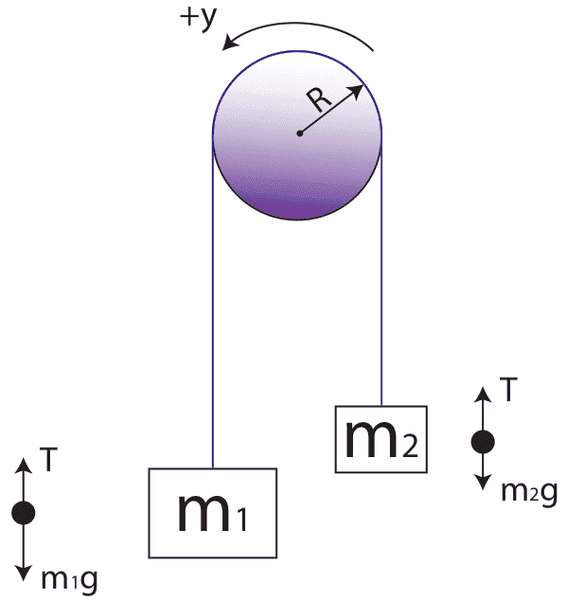# Coefficient of Friction of Fixed Atwood Pulley

• DrewCrew
In that case, you would either need to add more mass to the system to make it stable or find a way to increase the friction.f

## Homework Statement

Hi, I was wondering how you would isolate for and calculate the coefficient of friction in an Atwood pulley, with one fixed pulley, with two masses hanging off of it on either side. There is no table or surface that the masses are touching or resting on, they are both free-hanging on either side of the pulley. There is no person applying a force to it, the pulley is free-standing. No values are given, I am just trying to make a general equation for the coefficient of friction. A general diagram of the situation is:## Homework Equations

I know that, for m1, Fnet = Force of tension - Force of Gravity - Force of Friction, if it were to set "up" as the positive direction
And for m2, Fnet = Force of Gravity - Force of tension - force of friction
Force of friction = μ X Force normal
Feel free to correct me if I'm wrong for any of these equations

## The Attempt at a Solution

Fnet1 = Ft - Fg - μFn
m1a = Ft - mg - μFn
In this case, would Fn be equivalent to Fg?
m1a = Ft - mg - μmg
μmg = Ft - mg - m1a
μ = (Ft - mg - m1a) / mg

I am attempting this entirely off of assumptions, as I am not aware of the actual friction forces or formulas on the pulley. Thanks for the help, it is greatly appreciated!

What friction are you considering?
Most problems involving pulleys tell you to assume the pulley is massless and "is frictionless". By that, they mean it has no frictional torque from its axle. Generally you should assume there is enough friction between the pulley and rope that the rope does not slip on the pulley, but if the pulley is massless then even the tiniest static friction will achieve that, so there is no way to determine what the coefficient is.

It gets a bit more interesting if the pulley has mass, and hence a moment of inertia. In every such problem I have ever seen posed, you are still to assume the rope does not slip on the pulley. If the pulley is to accelerate then there has to be enough friction to ensure there is no slippage, so you could calculate a minimum value for the static friction coefficient.

A problem could consider the case where the pulley has mass and the static friction is not enough to prevent slipping, but I have never seen that done.